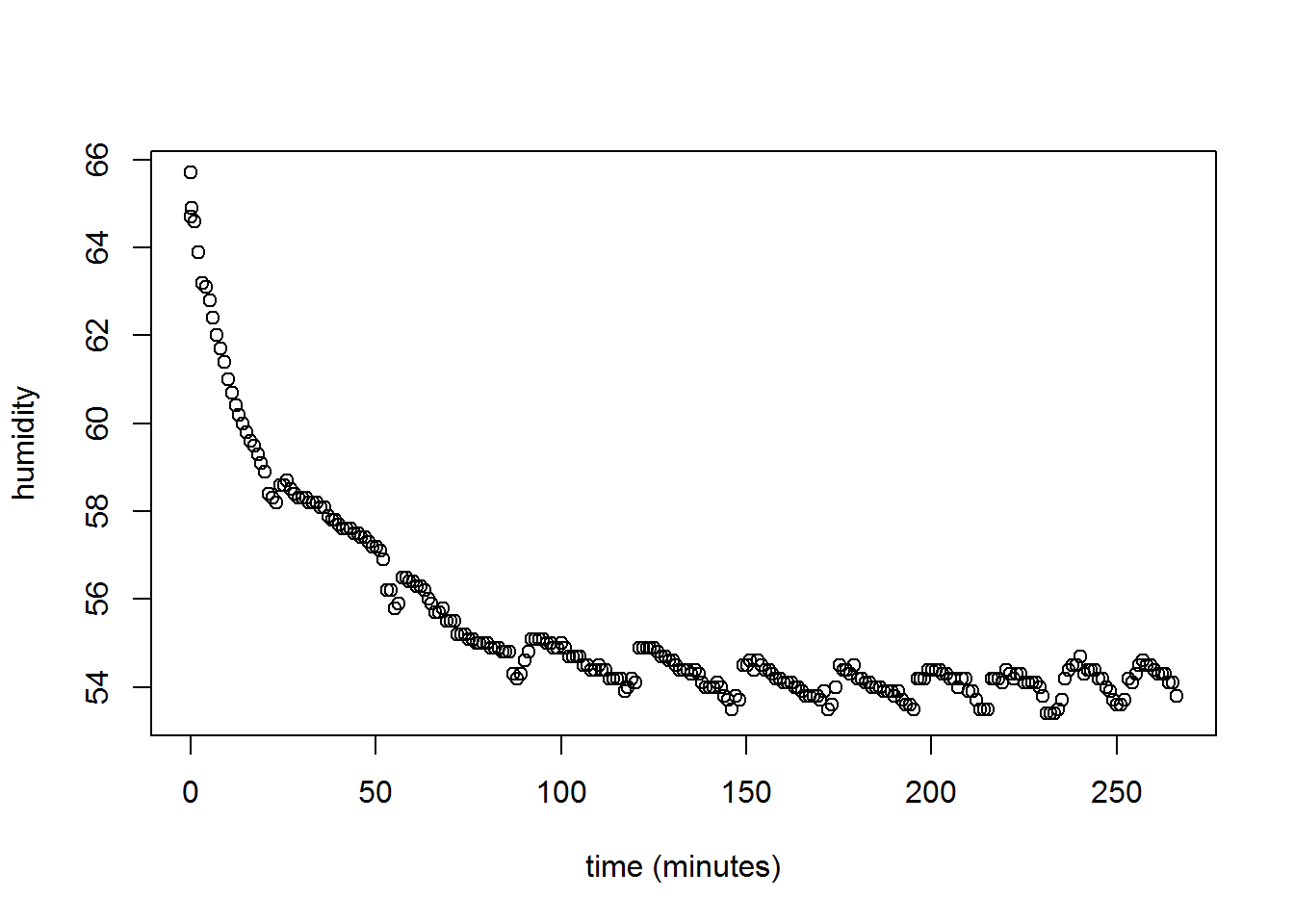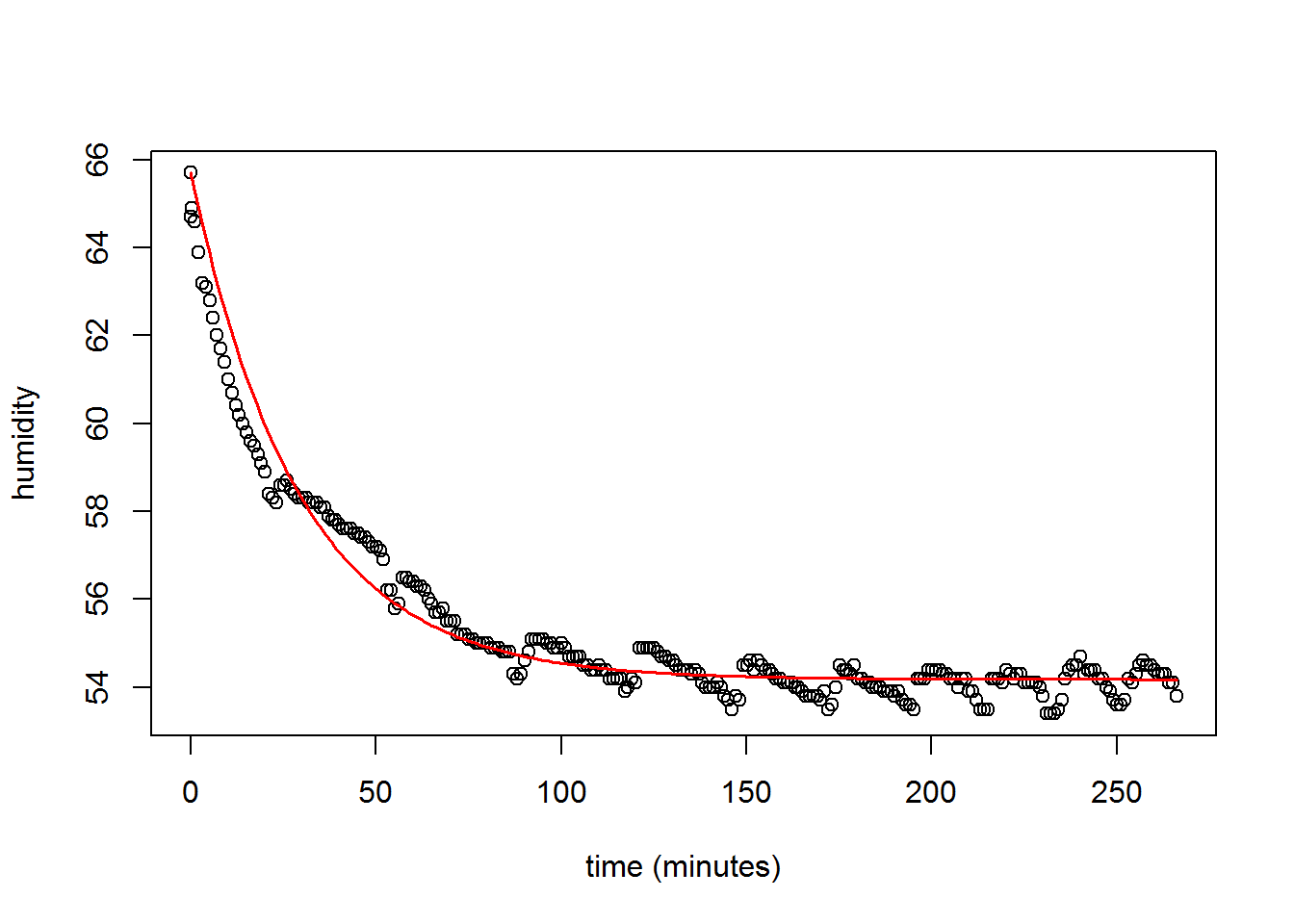My wife and I moved into a new house recently. We quickly discovered that the basement required a dehumidifier. We purchased one and hooked it up to a hose, and to the drain. Ideally, it will happily and indefinitely dehumidify without intervention. Occassionally, though, it doesn’t fully drain into the hose, the dehumidifier’s tank gets full, and the machine turns off. This morning the dehumidifier tank was full, so the machine was off and the humidity had risen. I emptied the tank and the machine faithfully restarted its job. I measure the humidity using a Raspberry Pi and a DHT22 sensor. So we can examine the data to see what this looks like quantitatively.

I first read in a limited dataset starting with approximately the point at which I drained the tank and the dehumidifier restarted.

## Load the data
d <- read.csv("humid.csv")

# recenter time and convert to minutes
d$time <- (d$time - min(d$time)) / 60 For what it’s worth, the measurement device was on the opposite side of the room relative to the dehumidifier. Below I plot the data. plot(d$time, d$humid, ylab="humidity", xlab="time (minutes)")Just by the way the data look, I can probably model this as an exponential decay. It appears that the change in humidity is some function of the humidity level itself. In other words, the ease with which the dehumidifier can pull moisture out of the air probably depends on how much moisture is in the air. This makes pretty good sense even without seeing the data. So I’ll fit a model that looks like this: $h = \beta + (h_0 - \beta) e^{-\lambda t}$ In which $$\beta$$ is a floor humidity level (the lowest it appears to go) that is estimated from the data, $$\lambda$$ represents the speed of “decay” towards the floor, $$t$$ is time in minutes, $$h$$ is the humidity level, and $$h_0$$ is the initial humidity level (65.7%). ans <- nls(humid ~ floor + (65.7-floor)*exp(-1*lambda*time), data=d, start=c(floor=10, lambda=2)) summary(ans) ## ## Formula: humid ~ floor + (65.7 - floor) * exp(-1 * lambda * time) ## ## Parameters: ## Estimate Std. Error t value Pr(>|t|) ## floor 5.418e+01 4.086e-02 1326.12 <2e-16 *** ## lambda 3.439e-02 6.754e-04 50.91 <2e-16 *** ## --- ## Signif. codes: 0 '***' 0.001 '**' 0.01 '*' 0.05 '.' 0.1 ' ' 1 ## ## Residual standard error: 0.5131 on 267 degrees of freedom ## ## Number of iterations to convergence: 9 ## Achieved convergence tolerance: 2.467e-06 For the moment, I will ignore the coefficient estimates. Below I replot the points and add a line that represents the fitted model. plot(d$time, d$humid, ylab="humidity", xlab="time (minutes)") lines(d$time, coef(ans) + (65.7-coef(ans))*exp(-1*coef(ans)*d\$time), col='red', lwd=1.5)The model appears to be a reasonable approximation of the data. The good fit suggests we might be able to use estimates of $$\beta$$ and $$\lambda$$ for data collected at various points in the basement to learn how well a fixed-position dehumidifier can dehumidify the entire basement. For example, if $$\lambda$$ ends up being larger when we collect data much closer to the dehumidifier, then we’ll have a sense for how dehumidification speed depends on distance from the dehumidifier. Likewise, if the floor ($$\beta$$) changes substantially according to distance from the dehumidifier, we may have an even more direct reason to reposition the dehumidifier to a more central location. If it doesn’t, or if it doesn’t change enough to matter (e.g., 1 or 2 percentage points), then it’s probably OK where it is—in a corner out of the way.

A second take-home is that once the humidity hits the floor, it continually oscillates up and down (see plot). This could be the result of lots of things, but I suspect it represents a battle between the dehumidifier and the whole-house humidifier attached to our HVAC system. It could also simply be the humidistat kicking it on and off; however, I know it’s not that because we’ve set it much lower than 55%. I want to start experimenting with the whole-house humidifier by turning it down a notch to see if the floor changes or if the oscillations stop.